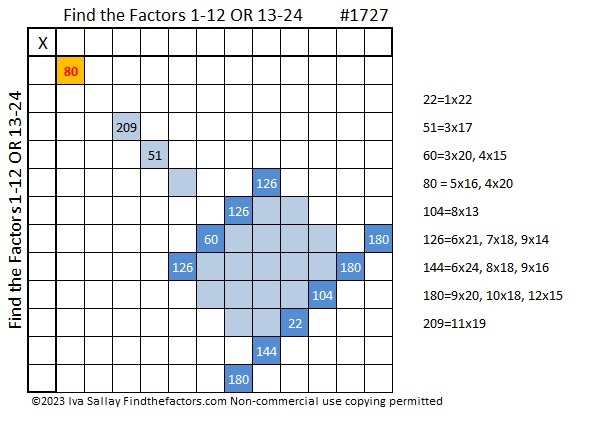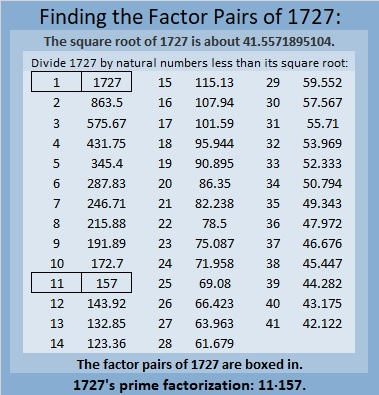# 1727 A Happy Birthday Cake for Jo Morgan

Contents

### Today’s Puzzle:

Today is Jo Morgan’s birthday, so I made her a cake!

Use logic to find the factors in the cake. There is only one solution. Yesterday’s puzzle was pretty easy at least to get started. This one won’t be. You’ll have to use logic just to figure out which set of numbers belongs in the top row, 1-12 OR 13-24, and which set belongs in the first column. Not only that but both 126 and 180 appear THREE times in the puzzle! Have a party figuring it out!### Factors of 1727:

1 – 7 + 2 – 7 = -11, a multiple of 11, so 1727 is divisible by 11.

• 1727 is a composite number.
• Prime factorization: 1727 = 11 × 157.
• 1727 has no exponents greater than 1 in its prime factorization, so √1727 cannot be simplified.
• The exponents in the prime factorization are 1 and 1. Adding one to each exponent and multiplying we get (1 + 1)(1 + 1) = 2 × 2 = 4. Therefore 1727 has exactly 4 factors.
• The factors of 1727 are outlined with their factor pair partners in the graphic below.### More About the Number 1727:

1727 is the hypotenuse of a Pythagorean triple:
935-1452-1727, which is 11 times (85-132-157).

From OEIS we learn that
1727 = 12³ – 1³, and
7271 = 20³ – 9³.
Thus 1727 forward and backward is the difference of two cubes!

## 2 thoughts on “1727 A Happy Birthday Cake for Jo Morgan”

1.Paula B Krieg

1727 is a thoroughly delightful number to start my day with.

•ivasallay

Thank you. I’m very happy you came here to enjoy it!

This site uses Akismet to reduce spam. Learn how your comment data is processed.